# 戒指 橙色传奇装备 - 火炬之光无限

icon affix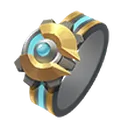机械之心

(25–30) 最大生命

+10% 移动速度
+10% 攻击速度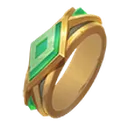谎言

(25–30) 最大魔力

+10% 移动速度
+10% 施法速度火焰降生

(16–24)% 火焰抗性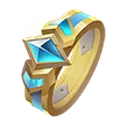黑云之簇

(30–40)% 闪电伤害
(30–50) 最大生命
(30–40)% 暴击值
(-40–-30)% 暴击伤害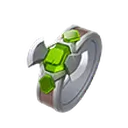狂怒之心

(25–35) 敏捷
(8–10)% 攻击速度
(25–35)% 攻击暴击伤害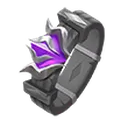号令指环

(20–40)% 魔力自然回复速度
(50–60)% 召唤物伤害冰结雷光

(15–25) 点敏捷与智慧
(40–50)% 元素伤害
(12–16)% 冰冷与闪电抗性
50% 闪电伤害转化为冰冷伤害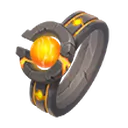万界

(10–15)% 最大护盾
(10–15)% 最大生命
(12–16)% 腐蚀抗性破厄雷狱

(20–30)% 闪电抗性
(20–25)% 触电几率

-25%雷霆加持魔力封印

(-50–-60)% 光环效果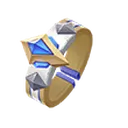元素使者

(30–40)% 元素伤害
(14–18)% 元素抗性
(20–30)% 几率造成元素异常状态
(20–30)% 几率避免元素异常凋零指环

(50–60) 最大生命
(20–30) 最大护盾
(30–40)% 持续伤害
(10–50) 每秒施加加剧
-30% 技能范围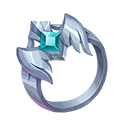风息之引

(60–80) 最大生命
(60–80) 最大护盾
(15–20)% 元素抗性
-20% 移动速度风息之散

(60–80) 最大生命
(60–80) 最大护盾
(15–20)% 元素抗性
-20% 冷却回复速度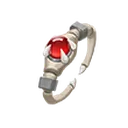灼热指骨

(30–40)% 火焰抗性
+25% 点燃几率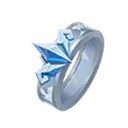冰山旅人

(20–25)% 火焰与冰冷抗性
(30–40)% 冰结几率
50% 物理伤害转化为冰冷伤害
(40–60)% 寒冰加持的增益效果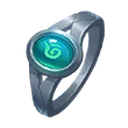元素之雨

(100–120) 最大生命
(30–36)% 护甲值
(30–36)% 近战伤害清净幻痛

(45–50)% 持续伤害
(12–20)% 技能效果持续时间

(12–15)% 火焰与冰冷抗性极寒之环

(25–35)% 冰冷抗性

50% 物理伤害转化为冰冷伤害
+50% 冰结几率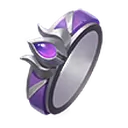哀嚎指轮

(10–12)% 攻击速度
(30–50)% 投射物伤害
(30–50)% 投射物速度

+1 抛射投射物分裂数量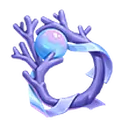永眠者的拥怀

(16–20)% 防御值
(6–9)% 护盾返还

+50% 几率避免异常状态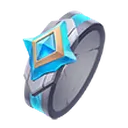躁动冰河

(8–12)% 最大魔力
(45–55) 智慧
(35–40)% 冰冷抗性喑腐神智

(-8–-6)% 魔力封印
(12–15)% 元素抗性
+1 智械召唤物数量上限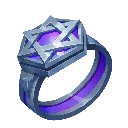混乱终焉

(20–30) 全属性
(8–10)% 最大生命
(8–10)% 最大护盾
<随机一条六色增益词缀>
<随机一条六色增益词缀>
<随机一条六色增益词缀>不燃之火

(8–12)% 最大生命和最大护盾

(40–60)% 攻击伤害

(25–35)% 火焰与闪电抗性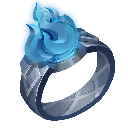不冻之冰

(8–12)% 最大生命和最大护盾

(40–60)% 法术伤害

(25–35)% 冰冷与腐蚀抗性吉玛的渴望

(16–24)% 火焰与冰冷抗性